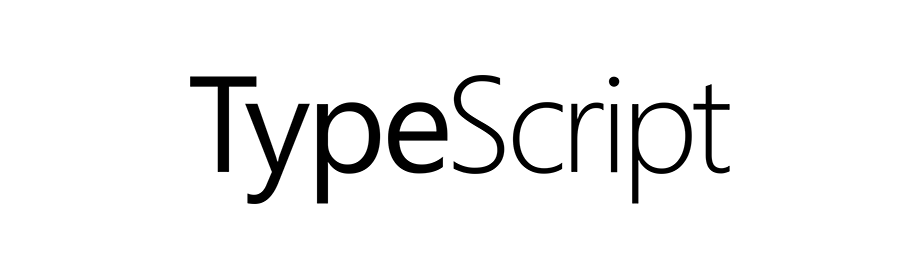# TypeScript - Alias for custom types

TypeScriptIn this tutorial we will learn to create alias for custom types in TypeScript.

In the previous tutorials we learned about functions and objects and we also covered arrow functions and also created custom types.

## Create a custom type

In the following example we are creating a variable of custom type.

``````let sampleUser: { username: string, points: number };
``````

So, we have a variable `sampleUser` of object type. The object type has two properties. The first one is `username` of type `string` and the second one is `points` of type `number`.

We can now assign a value to the `sampleUser` variable that matches the above type.

In the following example we are assigning a value to the `sampleUser` variable.

``````// declare variable and type
let sampleUser: { username: string, points: number };

// now assign value
sampleUser = {
points: 10
};
``````

## Create an alias for custom type

Following is the syntax to create an alias for custom type.

``````type aliasName = customType;
``````

Where, `type` is a keyword to create an alias. The name of the alias is `aliasName` and it is an alias for the given custom type denoted by `customType`.

## Example: Alias for object type

So, in the above example we have a custom type for the `sampleUser` variable.

``````{ username: string, points: number }
``````

We can create an alias for the above type as follows.

``````type UserData = { username: string, points: number };
``````

Now, we can use the new type `UserData` to create variables.

``````// creating a variable of type UserData
let sampleUser: UserData;
``````

And we can now assign a value to the variable `sampleUser` of type `UserData`.

``````// assign value
sampleUser = {
points: 10
};
``````

The final code for the above custom type will look like the following.

``````// custom type
type UserData = { username: string, points: number };

// creating a variable of type UserData
let sampleUser: UserData;

// assign value
sampleUser = {
points: 10
};

// output
console.log(sampleUser);
``````

Lets take one more example of custom type and this time we will create a function type.

## Example: Alias for function type

In the following example we are creating an alias for a custom function type.

``````type myFunction = (x: number, y: number) => number;
``````

So, the alias is `myFunction`. We are using arrow function to define the function type.

Looking at the function type `(x: number, y: number) => number` we can tell that the function will take two parameters of type `number` and will return value of type `number`.

Now, we will create a variable using the alias `myFunction`.

``````let addNumbers: myFunction;
``````

So, `addNumbers` is a variable that is of `myFunction` type. This means, we can only assign functions to the `addNumbers` variable that takes two parameters of `number` type and returns `number` value.

Now, we assign a function to the above variable.

``````addNumbers = function(a: number, b: number): number {
return a + b;
};
``````

The final code will look like the following.

``````// custom function type
type myFunction = (x: number, y: number) => number;

// create variable using custom type alias